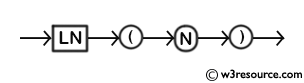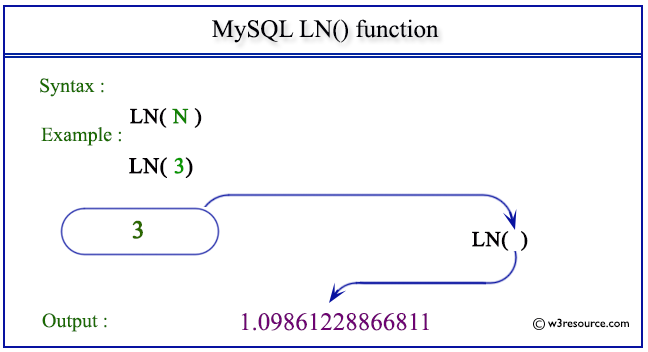# MySQL LN() function

## LN() function

MySQL LN() returns the natural logarithm of a number that is the base e logarithm of the number. The return value will be NULL when the value of the number is less than or equal to 0.

The EXP() is the inverse of this LN().

Syntax:

```LN (N);
```

Where N is a number.

Syntax Diagram:MySQL Version: 5.6

Pictorial presentation of MySQL IN() functionExample of MySQL IN() function

Code:

``````SELECT LN(3);
```
```

Explanation

The above MySQL statement returns the natural logarithm of 3.

Sample Output:

```mysql> SELECT LN(3);
+------------------+
| LN(3)            |
+------------------+
| 1.09861228866811 |
+------------------+
1 row in set (0.00 sec)
```

Example : LN() function with negative value

Code:

``````SELECT LN(-3);
```
```

Explanation

The above MySQL statement returns NULL because the value specified in the argument is less than 0.

Sample Output:

```mysql> SELECT LN(-3);
+--------+
| LN(-3) |
+--------+
|   NULL |
+--------+
1 row in set (0.00 sec)
```

All Mathematical FunctionsPrevious: FLOOR()
Next: LOG()

﻿